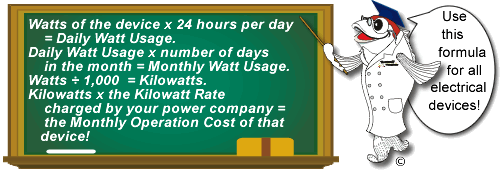# What is the Electrical Power Costs of my Pond Pump?## How To Calculate theMonthly Operation Costof Pumps and other electrical devices

The formula is simple. Somewhere on your pump or electrical device the wattage should be listed. If not, you can calculate the wattage if the Amps and Volts are listed. (Amps x Volts = Watts)

Your local electrical company charges you by the "Kilowatt Hour". So to accurately measure the Monthly Operational Cost of any electrical device, it is important to start with its wattage.

Watts x 24 = The total Watts used in a 24 hour period - or "Daily Watts".

Next, multiply the "Daily Watts" by the number of days in the month. This will be the "Monthly Watts".

Divide the Monthly Watts by 1,000 to convert the Monthly Watts into Monthly Kilowatts.

Multiply the Monthly Kilowatt by the price your local power company charges per Kilowatt. That's it! You now know how to calculate the monthly power cost of any electrical device.

You can also calculate the Monthly Operational Cost of a device by first converting the wattage to Kilowatts like this:

Multiply the watts x 24 hours, then divide by 1000 for kilowatts.  Multiply the total by your price per kilowatt hour which will equal your daily cost.  Then multiply the number of days in the month to get your monthly total.  Example;  a 115 volt, 2.8 amp, 322 watt pump.To figure the monthly operation cost of this pump;  322 watts X 24 hours ÷ by 1000 kilowatts = 7.72 kilowatts per day the pump is consuming. Multiply 7.72 kilowatts by your kilowatt cost (in our case it is \$0.08 per kilowatt hour) to get the daily cost of running the pump.  7.72 x \$0.08 = \$0.62 per day to run that pump.  Multiply the daily rate by the number of days in the month, 30 for example, and you get the monthly operation cost.

\$0.62 per day X 30 days = \$18.60 per month to operate this pump!

Once again, the formula is:

watts X 24 hours ÷ 1000 kilowatts X kilowatt cost X days in the month or
amps X volts X 24 hours ÷ 1000 kilowatts X kilowatt cost X days in the month

Top of Page